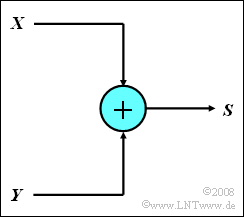# Exercise 1.1Z: Sum of Two Ternary SignalsSum $S$ of two
ternary signals  $X$  and  $Y$

Let two three-stage message sources  $X$  and  $Y$  be given,  whose output signals can only assume the values  $-1$,  $0$  and  $+1$  respectively.  The signal sources are statistically independent of each other.

• A simple circuit now forms the sum signal  $S = X + Y$.
• At the signal source  $X$,  the values  $-1$,  $0$  and  $+1$  occur with equal probability.
• For source  $Y$,  the signal value  $0$  is twice as likely as the other two values  $-1$  and  $+1$, respectively.

Hints:

• Solve the subtasks  (3)  and  (4)  according to the classical definition.
• Nevertheless,  consider the different occurrence frequencies of the signal  $Y$.
• The topic of this section is illustrated with examples in the (German language) learning video
Klassische Definition der Wahrscheinlichkeit  $\Rightarrow$ "Classical definition of probability".

### Questions

1

What are the probabilities of occurrence of the signal values of  $Y$?  What is the probability that  $Y = 0$ ?

 ${\rm Pr}(Y=0) \ = \$

2

How many different signal values  $(I)$  can the sum signal  $S$  assume?  Which are these?

 $I \ = \$

3

What are the probabilities of the values determined in subtask  (2)?  How probable is the maximum value  $S_{\rm max}$?

 ${\rm Pr}(S = S_{\rm max} ) \ = \$

4

How do the probabilities change,  if now instead of the sum the difference  $D = X - Y$  is considered?  Give reasons for your answer.

 The probabilities remain the same. The probabilities change.  How do they change?

### Solution

#### Solution

(1)  Since the probabilities of  $\pm 1$  are the same and  ${\rm Pr}(Y = 0) = 2 \cdot {\rm Pr}(Y = 1)$  holds, we get:

$${\rm Pr}(Y = 1) + {\rm Pr}(Y = 0) + {\rm Pr}(Y = -1) = 1/2 \cdot {\rm Pr}(Y = 0) + {\rm Pr}(Y = 0) + 1/2\cdot {\rm Pr}(Y = 0) = 1\hspace{0.3cm} \Rightarrow \hspace{0.3cm}{\rm Pr}(Y = 0)\;\underline { = 0.5}.$$

(2)  $S$  can take a total of  $\underline {I =5}$  values, namely  $0$,  $\pm 1$  and  $\pm 2$.

(3)  Since  $Y$  is not equally distributed, one cannot (actually) apply the "Classical Definition of Probability" here.

• However,  if we divide  $Y$  into four ranges according to the graph,  assigning two of the ranges to the event  $Y = 0$,  we can still proceed according to the classical definition.
• One then obtains:
$${\rm Pr}(S = 0) = {4}/{12} = {1}/{3},$$
$${\rm Pr}(S = +1) = {\rm Pr}(S = -1) ={3}/{12} = {1}/{4},$$
$${\rm Pr}(S = +2) = {\rm Pr}(S = -2) ={1}/{12}$$
$$\Rightarrow \hspace{0.3cm}{\rm Pr}(S = S_{\rm max}) = {\rm Pr}(S = +2) =1/12 \;\underline {= 0.0833}.$$

(4)  It is also evident from the graph that the difference signal  $D$  and the sum signal  $S$  take the same values with equal probabilities.

• This was to be expected,  since  ${\rm Pr}(Y = +1) ={\rm Pr}(Y = -1)$  is given   ⇒   Proposed solution 1.